# Truncated cuboctahedron

Truncated cuboctahedron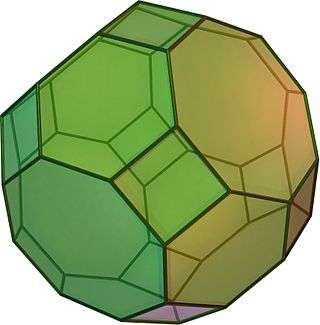TypeArchimedean solid
Uniform polyhedron
ElementsF = 26, E = 72, V = 48 (χ = 2)
Faces by sides12{4}+8{6}+6{8}
Conway notationbC or taC
Schläfli symbolstr{4,3} ort0,1,2{4,3}
Wythoff symbol2 3 4 |
Coxeter diagramSymmetry groupOh, B3, [4,3], (*432), order 48
Rotation groupO, [4,3]+, (432), order 24
Dihedral Angle4-6: arccos(−6/3) = 144°44′08″
4-8: arccos(−2/3) = 135°
6-8: arccos(−3/3) = 125°15′51″
ReferencesU11, C23, W15
PropertiesSemiregular convex zonohedron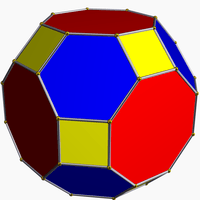Colored faces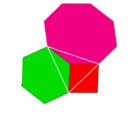4.6.8
(Vertex figure)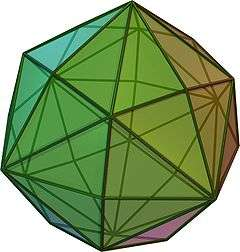Disdyakis dodecahedron
(dual polyhedron)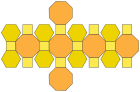Net

In geometry, the truncated cuboctahedron is an Archimedean solid. It has 12 square faces, 8 regular hexagonal faces, 6 regular octagonal faces, 48 vertices and 72 edges. Since each of its faces has point symmetry (equivalently, 180° rotational symmetry), the truncated cuboctahedron is a zonohedron.

## Other names

Alternate interchangeable names are:

The name truncated cuboctahedron, given originally by Johannes Kepler, is a little misleading. If you truncate a cuboctahedron by cutting the corners off, you do not get this uniform figure: some of the faces will be rectangles. However, the resulting figure is topologically equivalent to a truncated cuboctahedron and can always be deformed until the faces are regular.

The alternative name great rhombicuboctahedron refers to the fact that the 12 square faces lie in the same planes as the 12 faces of the rhombic dodecahedron which is dual to the cuboctahedron. Compare to small rhombicuboctahedron.

One unfortunate point of confusion: There is a nonconvex uniform polyhedron by the same name. See nonconvex great rhombicuboctahedron.

## Cartesian coordinates

The Cartesian coordinates for the vertices of a truncated cuboctahedron having edge length 2 and centered at the origin are all permutations of:

(±1, ±(1 + 2), ±(1 + 22))

## Area and volume

The area A and the volume V of the truncated cuboctahedron of edge length a are:## Dissection

The truncated cuboctahedron can be dissected into a central rhombicuboctahedron, with 6 square cupolas above each primary square face, 8 triangular cupolas above each triangular face, and 12 cubes above the secondary square faces.

A dissected truncated cuboctahedron can create a genus 5, 7 or 11 Stewart toroid by removing the central rhombicuboctahedron and either the square cupolas, the triangular cupolas or the 12 cubes respectively. Many other lower symmetry toroids can also be constructed by removing a subset of these dissected components. For example, removing half of the triangular cupolas creates a genus 3 torus, which (if they are chosen appropriately) has tetrahedral symmetry.

Stewart toroids
Genus 3 Genus 5 Genus 7 Genus 11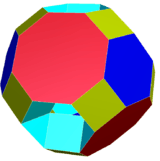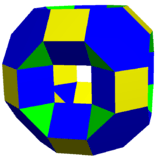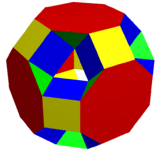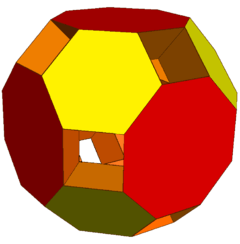## Uniform colorings

There is only one uniform coloring of the faces of this polyhedron, one color for each face type.

A 2-uniform coloring, with tetrahedral symmetry, exists with alternately colored hexagons.

## Orthogonal projections

The truncated cuboctahedron has two special orthogonal projections in the A2 and B2 Coxeter planes with  and  projective symmetry, and numerous  symmetries can be constructed from various projected planes relative to the polyhedron elements.

Orthogonal projections
Centered by Vertex Edge
4-6
Edge
4-8
Edge
6-8
Face normal
4-6
Image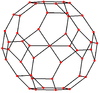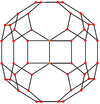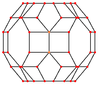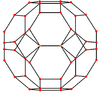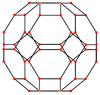Projective
symmetry
+    
Centered by Face normal
Square
Face normal
Octagon
Face
Square
Face
Hexagon
Face
Octagon
Image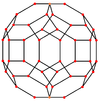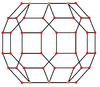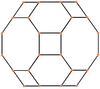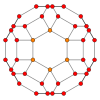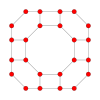Projective
symmetry
    

## Spherical tiling

The truncated cuboctahedron can also be represented as a spherical tiling, and projected onto the plane via a stereographic projection. This projection is conformal, preserving angles but not areas or lengths. Straight lines on the sphere are projected as circular arcs on the plane.

 Orthogonal projection Stereographic projections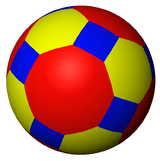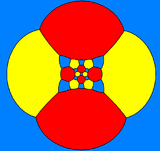square-centered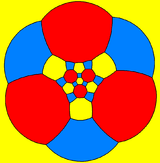hexagon-centered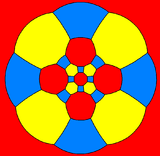octagon-centered

The truncated cuboctahedron is one of a family of uniform polyhedra related to the cube and regular octahedron.

This polyhedron can be considered a member of a sequence of uniform patterns with vertex figure (4.6.2p) and Coxeter-Dynkin diagram. For p < 6, the members of the sequence are omnitruncated polyhedra (zonohedrons), shown below as spherical tilings. For p < 6, they are tilings of the hyperbolic plane, starting with the truncated triheptagonal tiling.

## Truncated cuboctahedral graph

Truncated cuboctahedral graph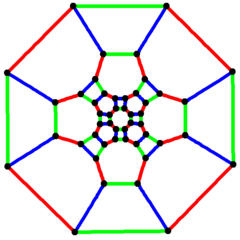4-fold symmetry
Vertices 48
Edges 72
Automorphisms 48
Chromatic number 2
Properties Cubic, Hamiltonian, regular, zero-symmetric

In the mathematical field of graph theory, a truncated cuboctahedral graph (or great rhombcuboctahedral graph) is the graph of vertices and edges of the truncated cuboctahedron, one of the Archimedean solids. It has 48 vertices and 72 edges, and is a zero-symmetric and cubic Archimedean graph.Wikimedia Commons has media related to Truncated cuboctahedron.